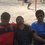# is this changing subject of formula possible? .... :X

If $y=x^{x^{x}}$

Make $x$ as the subject of formula. ..

ie. $x=f(y)$?

Is it possible to do so ?Note by Poonayu Sharma
6 years, 11 months ago

This discussion board is a place to discuss our Daily Challenges and the math and science related to those challenges. Explanations are more than just a solution — they should explain the steps and thinking strategies that you used to obtain the solution. Comments should further the discussion of math and science.

When posting on Brilliant:

• Use the emojis to react to an explanation, whether you're congratulating a job well done , or just really confused .
• Ask specific questions about the challenge or the steps in somebody's explanation. Well-posed questions can add a lot to the discussion, but posting "I don't understand!" doesn't help anyone.
• Try to contribute something new to the discussion, whether it is an extension, generalization or other idea related to the challenge.
• Stay on topic — we're all here to learn more about math and science, not to hear about your favorite get-rich-quick scheme or current world events.

MarkdownAppears as
*italics* or _italics_ italics
**bold** or __bold__ bold
- bulleted- list
• bulleted
• list
1. numbered2. list
1. numbered
2. list
Note: you must add a full line of space before and after lists for them to show up correctly
paragraph 1paragraph 2

paragraph 1

paragraph 2

[example link](https://brilliant.org)example link
> This is a quote
This is a quote
    # I indented these lines
# 4 spaces, and now they show
# up as a code block.

print "hello world"
# I indented these lines
# 4 spaces, and now they show
# up as a code block.

print "hello world"
MathAppears as
Remember to wrap math in $$ ... $$ or $ ... $ to ensure proper formatting.
2 \times 3 $2 \times 3$
2^{34} $2^{34}$
a_{i-1} $a_{i-1}$
\frac{2}{3} $\frac{2}{3}$
\sqrt{2} $\sqrt{2}$
\sum_{i=1}^3 $\sum_{i=1}^3$
\sin \theta $\sin \theta$
\boxed{123} $\boxed{123}$

## Comments

Sort by:

Top Newest

Because it is invertible, It might be possible.

- 6 years, 11 months ago

Log in to reply

x will always remain one and it is independant of y

- 6 years, 11 months ago

Log in to reply

I don't think it can x can be expressed by y in elemantary form, such as polynomials, trigonometry etc.In the case $x^x=y$, the solutions for x need the Lambert W function, which is kinda weird, because it's just defined to be the solution to $x.e^x=y$, and it cannot be expressed with elementary functions. Maybe someone who knows about it can make a note or something.In the case $x^{x^x}$, I haven't the vaguest idea.

- 6 years, 11 months ago

Log in to reply

I meant x=y root y

- 6 years, 11 months ago

Log in to reply

Can u explain?

- 6 years, 11 months ago

Log in to reply

the question is y=x to the power x and this continues till infity.Now if you remove 1 unit from infinity then also it remains infinity only.Hence you write y=x to the power y.

- 6 years, 11 months ago

Log in to reply

It is not till infinity but only 2 consecutive powers of x and if we take an example ...

16= 2^2^2..

by ur method, we get 2=16^(1/16) 16=2^16.(in 2nd reply) ..which is incorrect

- 6 years, 11 months ago

Log in to reply

I edited to reflect the author's intentions. Check again now.

- 6 years, 11 months ago

Log in to reply

I didnt want the powers to go till infinity. ..but only 2 powers ..sorry to not notice your edition...but changing the subject to x with this condition, is possible?

- 6 years, 11 months ago

Log in to reply

Are you a moderator ?

- 6 years, 11 months ago

Log in to reply

If you solved it considering it going to infinity then it seems correct

- 6 years, 11 months ago

Log in to reply

×

Problem Loading...

Note Loading...

Set Loading...